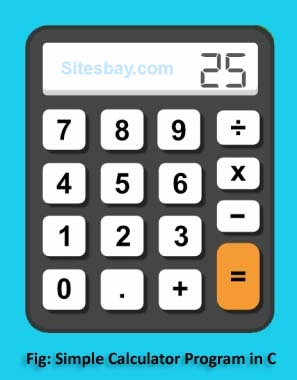# Calculator Program in C

## Write Simple Calculator Program in C

In C language you can add, subtract, multiply, divide any number, these all operation you can perform by using switch case.Calculators are widely used device nowadays. It makes calculations easier and faster. Calculators are used to everyone in daily life. A simple calculator can be made using a C program which is able to add, subtract, multiply and divide, two operands entered by the user. The switch and break statement is used to create a calculator.

## Calculator Program in C

```#include<stdio.h>
#include<conio.h>

void main()
{
char choice;
int a,b,res=0;
clrscr();
printf("Enter First value: ");
scanf("%d",&a);
printf("\n Enter Operator: ");
choice=getch();
printf("\n Enter Second value: ");
scanf("%d",&b);

switch(choice)
{
case '+':
res=a+b;
printf("Sum: %d",res);
break;
case '-':
res=a-b;
printf("Difference: %d",res);
break;
case '*':
res=a*b;
printf("Product: %d",res);
break;
case '/':
res=a/b;
printf("Quotient: %d",res);
break;
default:
printf("Enter Valid Operator!!");
}
getch();
}
```

## Output

```Enter First number: 6
Enter operator : +
Enter Second number: 10
Sum: 16
```

Explanation: In above code choice=getch(); is used for scan or get one value by using getch() function from keyboard, and store in choice.

WIFI CCTV Camera